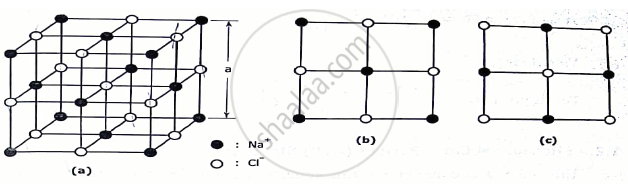Advertisement Remove all ads

# With neat diagram of unit cell, explain the structure of NaCl crystal and calculate the no .of ions per unit cell, co ordination no. and lattice constant. - Applied Physics 1

Answer in Brief

With neat diagram of unit cell, explain the structure of NaCl crystal and calculate the no .of ions per unit cell, co ordination no. and lattice constant. Calculate the packing factor of NaCl crystal assuming the radius of Na+is 0.98 A° and radius of Cl is 1.81 A°.

Advertisement Remove all ads

#### Solution

NaCl STRUCTURE:-

This is an ionic structure in which the Na+ions and Cl
ions are alternately arranged. It is a combination of two FCC sublattice one made up of Na+ions and the other of Cl ions as if one sublattice is translated through the other along the cube edges.

NaCl unit cell with Na+ ions occupying the regular FCC lattice points with Cl ions positioned at alternate points. A face of this unit cell is shown.
Another NaCl unit cell can be considered with the positions of Na+ and Cl ions interchanged. The face of such a unit cell is shown.
NaCl UNIT CELL PARAMETER:⦁ Total number of molecule / unit cells
Calculation for Na+ = Here Na+ forms a FCC structure. Hence total number of Na+ions = 4

Calculation for Cl = There are 12Cl  ions at the edges. Every edge lattice points is shared by four neighbouring unit cell. Hence every edge lattice point carries ¼ of an atom. There is one whole Cl  ion at the centre of the structure. Hence ,

Total number of Cl ions = (12 ×1/4) + 1 = 4.

Since there are 4 Na+ ions and four Cl ions in a NaCl unit cell , there are four NaCl molecule  present in a unit cell.
Hence number of molecule / unit cell = 4.
⦁ Atomic Radius (r)
Since NaCl is an ionic structure and cations are smaller than anions it is assumed that radius of cation = rc and the radius of an anion = rA

⦁ Atomic packing factor(APF)

APF =((4xx4/3πr_c^3) xx(4xx4/3πr_A^3))/(a^3)    it is found that a = 2r_c + 2r_A

Hence, APF=((2π)/3)(r_c^3 + r_A^3)/(rC+rA)^3

(d) Void space .

This is given by[1-((2π)/3) (r_c^3 + r_A^3)/(rC +r
A)^3]

NUMERICAL:-

GivenData :-rC=0.98A°,  rA =1.81A°

Formula :-   APF= (2π)/3.(r_c^3+r_A^3)/(r_c+r_A)^3.

Calculations :- APF= (2π)/3(0.98^3+1.81^3)/(0.98+1.81)^3  .=0.66.

Answer :-  APF   =0.66

Concept: Study of characteristics of unit cell of Diamond, ZnS, NaCl and HCP;
Is there an error in this question or solution?
Advertisement Remove all ads

#### APPEARS IN

Advertisement Remove all ads
Advertisement Remove all ads
Share
Notifications

View all notifications

Forgot password?